# Geometry construction construct an equilateral triangle

##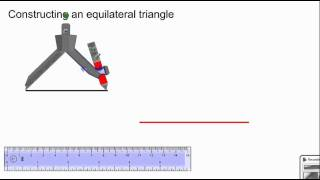By Soowook Lee

Construct an equilateral triangle.# Compass constructions 2

##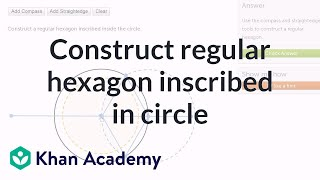By Khan Academy

Compass constructions 2# Constructing a line tangent to a circle

##By Khan Academy

Constructing a line tangent to a circle# Compass constructions 2

##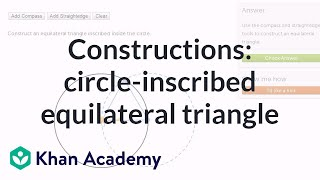By Khan Academy

Compass constructions 2# Compass constructions 2

##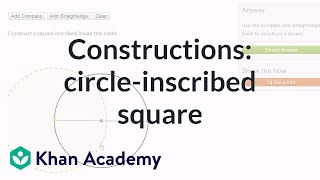By Khan Academy

Compass constructions 2# Compass constructions 1

##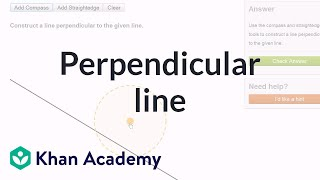By Khan Academy

Compass constructions 1# Constructing a line tangent to a circle

##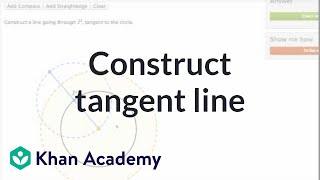By Khan Academy

Constructing a line tangent to a circle# Compass constructions 1

##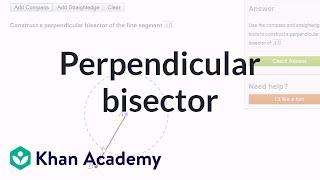By Khan Academy

Compass constructions 1# Compass constructions 1

##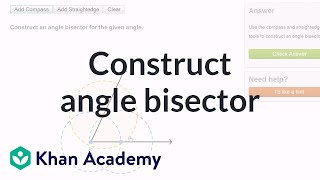By Khan Academy

Compass constructions 1# Construct A Square

##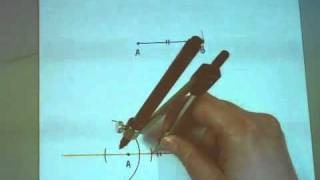By MrsBinomial

Another example of how to construct a square using a compass and straightedge.# Geometry Construction: Rotating a Figure by Hand

##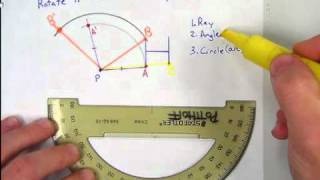By Matthew Potthoff

Geometry Construction: Rotating a Figure by Hand# Step-By-Step Instructions for Geometric Constructions

##By Ezlie

This video gives us each step of the essential geometric constructions. The first part of this video goes through some important geometric vocabulary (parallel lines midpoint etc) so you may want to skip ahead to 1:30 to get to the constructions. Constructions in this video: Copying a line segment Construcing the perpendicular bisector of a line segment Constructing a perpendicular to a line through a point not on the line. Constructing a perpendicular to a line through a point on the line. Bisecting an angle Copying an angle# Step-By-Step Instructions for Geometric Constructions

##By Ezlie

This video gives us each step of the essential geometric constructions. The first part of this video goes through some important geometric vocabulary (parallel lines, midpoint, etc), so you may want to skip ahead to 1:30 to get to the constructions. Constructions in this video: Copying a line segment Construcing the perpendicular bisector of a line segment Constructing a perpendicular to a line through a point not on the line. Constructing a perpendicular to a line through a point on the line. Bisecting an angle Copying an angle# Parallel Lines, Constructions, Transformations, Integers Quiz

##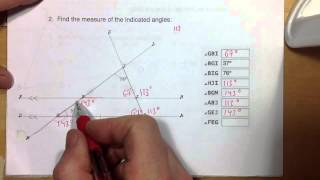By G Scruton

Parallel Lines, Constructions, Transformations, Integers Quiz# Geometry construction draw parallel lines

##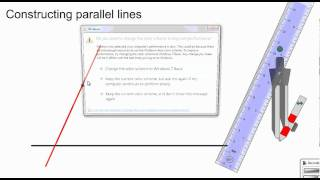By Soowook Lee

Youtube Presents Geometry construction draw parallel lines an educational video resources on math.# Constructing a Probability Distribution Table : ExamSolutions

##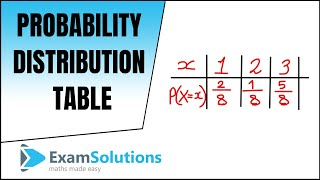By ExamSolutions

Youtube Presents Constructing a Probability Distribution Table : ExamSolutions an educational video resources on math.# Constructing polygon on the coordinate plane example

##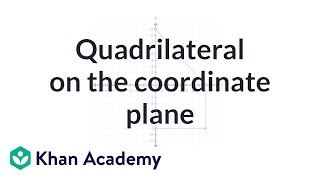By Khan Academy

In this example we are given the coordinates of the vertices and asked to construct the resulting polygon (specifically a quadrilateral). This is fun!# Understanding Quadrilaterals | Class 8th Mathematics | NCERT | CBSE Syllabus | Live Videos

##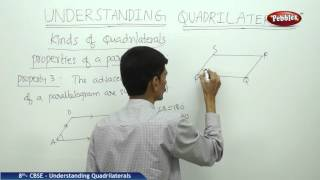By Pebbles CBSE Board Syllabus

Understanding Quadrilaterals | Class 8th Mathematics | NCERT | CBSE Syllabus | Live Videos This video is a part of Pebbles CBSE Syllabus Live Teaching Videos Pack.# Pyramids, prisms, cylinders and cones

##By MathPlanetVideos

Find the volume of a cone with the height 5 and the radius 3.# Proof: Rhombus diagonals are perpendicular bisectors | Quadrilaterals | Geometry | Khan Academy

##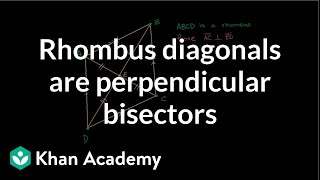By Khan Academy

Proving that the diagonals of a rhombus are perpendicular